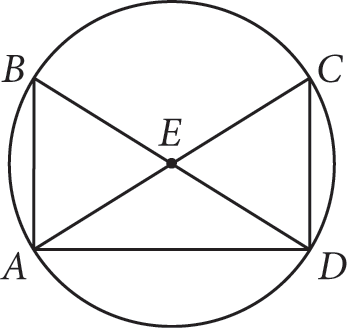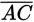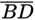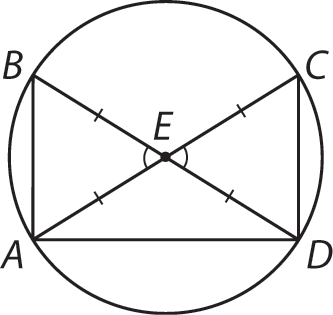# SAT Math Multiple Choice Question 964: Answer and Explanation

### Test Information

Question: 964

16.andare diameters of circle E above. Which triangle congruence theorem can be used to prove that ΔAEB is congruent to ΔDEC?

• A. AAA
• B. HL
• C. SAS
• D. SSA

Explanation:

C

Difficulty: Medium

Category: Additional Topics in Math / Geometry

Strategic Advice: Whenever you're asked to show that two triangles within a figure are congruent, mark up the figure to show which pairs of sides and which pairs of angles are congruent.

Getting to the Answer: Angles BEA and CED are vertical angles, and vertical angles are always congruent. Mark this on the diagram. Also, several sides of the triangles are radii, so they are all equal. Use hash marks to show this relationship. Your diagram should look something like this:Notice that the angles between the two sides with hash marks are congruent. This lets you demonstrate by SAS that ΔAEB ≅ ΔDEC, making (C) the correct answer.